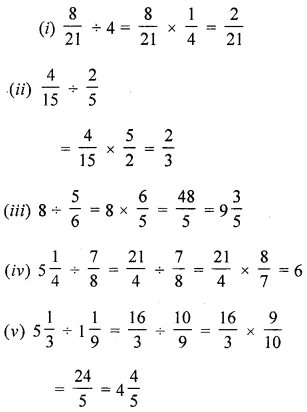# ML Aggarwal Class 6 Solutions for ICSE Maths Chapter 6 Fractions Ex 6.6

## ML Aggarwal Class 6 Solutions for ICSE Maths Chapter 6 Fractions Ex 6.6

Question 1.
Evaluate the following: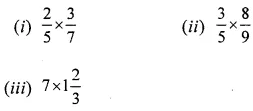Solution: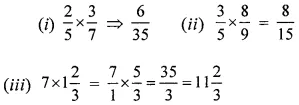Question 2.
Evaluate the following: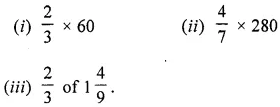Solution: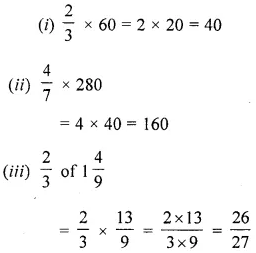Question 3.
Find the reciprocal of each of the following
(i) $$\frac{9}{13}$$
(ii) $$2 \frac{3}{8}$$
Solution: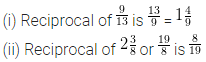Question 4.
Evaluate the following:Solution: